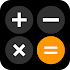# iOS 16 Calculator: iCalculator v2.1.9 (Mod Lite)

Free

### iOS 16 Calculator: iCalculator / Screenshots### iOS 16 Calculator: iCalculator / Description

iOS 16 Calculator: iCalculator app comes with a wide range of powerful features and a user-friendly interface for iOS 16! iCalculator – Scientific calculator has an all-in-one solution for all your mathematical needs. Whether you're a student professional or simply looking for a handy tool this iOS 16 Calculator has got you covered.

iOS Fraction Calculator is a powerful calculator tool that covers all your basic and advanced needs. Math Calculator performs simple calculations effortlessly with our user-friendly interface.

Need to solve complex equations or delve into scientific functions?

Scientific Calculator Free feature is specially developed for you. From logarithms to trigonometric functions Apple Calculator or Physics Calculator App offers a wide range of functions to meet your every mathematical requirement.

iOS 16 Calculator: iCalculator app not only act as Conversion Calculator and Currency Converter but it also acts like a Fractions Calculator Percentage Calculator Physics Calculator and Geometry Calculator. Simplify fractions and perform operations with ease using the fraction calculator. Calculate percentages discounts and interest rates quickly and accurately with Advanced Calculator App. Need to solve physics equations or perform geometry calculations? iCalculator's physics calculator and geometry calculator have the functionalities you need.

What sets iOS 16 Calculator: iCalculator app apart is its seamless integration with Apple devices. Simple Scientific Calculator App is designed exclusively for iOS and provides a smooth optimized experience that harnesses the full potential of Calculator For iPhone or iPad. Science Calculator is more than just an Android Calculator. Android Calculator is a perfect match for your iOS device complementing its sleek design and intuitive user interface.

Key Features of iOS 16 Calculator: iCalculator app:

• Fast and easy to use UI of Percentage Calculator Free App.
• Scientific Calculator solves complex equations.
• Perform mathematical functions with Math Calculator.
• Fraction Calculator simplifies fractions and performs operations accurately.
• Convert between different units with Conversion Calculator.
• Currency Converter Calculator effortlessly converts between currencies.
• Calculate percentages quickly and accurately via Percentage Calculator.
• Physics Calculator solves physics equations and performs calculations.
• Calculate geometric shapes angles and measurements with Geometry Calculator.
• Apple Calculator integrates with iOS devices for a seamless user experience.
• Access iPhone Calculator directly from home screen with Calculator Widget.
• Background and font color changing facility of Free Scientific Calculator App.
• Math Calculator keeps a record of all previous calculations in the history tab.
• Switch between different calculator modes as your specific needs in Multi Calculator.
• Simple Scientific Calculator provides a basic scientific calculator without compromising functionality.
• Advanced Calculator has advanced features such as logarithmic calculations trigonometric functions and more.

Download iOS 16 Calculator: iCalculator now and enjoy the convenience of having a powerful calculator currency converter and scientific tool all in one app.